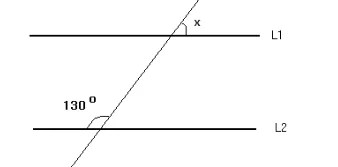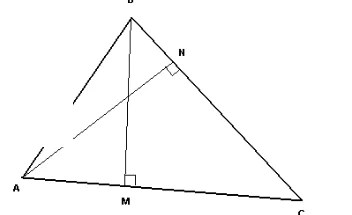Wednesday , September 29 2021
Breaking News

# NTS Quantitative Reasoning Practice Test 2

### NTS Quantitative Reasoning Practice Test # 2

INSTRUCTIONS:

• This Quiz is related to NTS Quantitative Reasoning Practice Test # 2 in this series
• The Quiz based on the Topic, “Quantitative Reasoning
• If you have good understanding about the subject, then you can attempt it
• There will be 10 Multiple Choice Questions (MCQs) in this test
• You have 60 Seconds to answer a single Multiple Choice Question
• Questions will be randomly changed every time you start this test
• You should practice more and more to get high marks
• Passing Criteria is 60 percent for this Quiz
• You can retake this test as many time as you like
• The progress bar at the top of screen will show your progress as well as the time remaining where timed quiz
• After the quiz, You will find Your Test Score and Grade
• If you feel that any Incorrect Answer to a Question, simply Comment us about the Question.

#### What is the measure of angle x if L1 and L2 are parallel lines?Correct! Wrong!

y + 130 = 180 or y = 180 - 130 = 50° x and y are vertical angles and therefore have equal measures x = 50°

#### If n is positive and less than 1, then which of the following is true? (I) n2 - n < 0 (II) n3 < n (III) n + 1 < 1

Correct! Wrong!

n is positive and less than 1 is translated as follows 0 < n < 1 Multiply all terms of the above inequality by n to obtain 0 < n2 < n which also gives n2 - n < 0 Hence statement (I) is true Multiply all terms of the above inequality by n to obtain 0 < n3 < n2 Since n2 < n, we have 0 < n3 < n Hence statement (II) is true For n = 0.75, statement (III) is not true Hence statement (I) and (II) only are true.

#### 8×2100 + 4×2101 =

Correct! Wrong!

Use the fact that 8 = 23 and 4 = 22 to rewrite the given expression as follows 8×2^100 + 4×2^101 = 23×2^100 + 22 ×2^101 Use rules of exponent to simplify = 2^103 + 2^103 = 2×2^103 = 2^104

#### The perimeter of a rectangle is 90 and its width is 10. What is its area?

Correct! Wrong!

Let L and W be the length and width of the rectangle. Using the formula of the perimeter, we write 2L + 2W = 90 W is given; hence 2L + 2(10) = 90 Solve for L 2L = 70 , L = 35 The area A of the rectangle is given by A = L * W = 35 * 10 = 350

#### What is the ratio of the area of a square of side x to the area of a rectangle of width 2 x and length 3 x? ​

Correct! Wrong!

The area of a square of side x is x2 and the area of a rectangle of width 2x and length 3x is (2 x)(3 x) = 6 x2. The ratio of the area of the square to the area of a rectangle is given by x2 / (6 x2) = 1 / 6

#### If p and q are integers, such that p<0<q, which of the following must be true? I. 2p<2q II. p2<q2 III. p+q=0 ​

Correct! Wrong!

Variables in the answer choices, plug in!! lets plug in p=-2 and q=2. Now verify all the choices given. We get II false. So all the answers which contain II should go. So (B), (C) and (E) go. Since two answer choices remain we plug in once more. Put p=-2 and q=3. Now we can see that III is false. So the answer is (A).

#### ABC is a triangle whose sides AB and BC have length of 3 and 5 respectively. Which of the following could be the length of side AC? (I) 8 (II) 12 (III) 0.5

Correct! Wrong!

The length of the third side of a triangle is always less than the sum and greater than the difference of the lengths of other two sides. The sum S and the difference D of the given sides is. S = 3 + 5 = 8 D = 5 - 3 = 2

#### What is X ?Correct! Wrong!

We don’t need to go about solving this question as per the REAL MATH way. We use our technique. Whenever we see variables in the answer choice we just plug in!! Plug in x=3,

#### ABC is a triangle where AN is perpendicular to CB and BM perpendicular to AC. The length of BC is 10, that of AC is 12 and that of AN is 8. Find the length of BM.Correct! Wrong!

The area A of the given triangle may be calculated using the two altitutdes as follows A = (1/2)(AN)(BC) or A = (1/2)(BM)(AC) Hence (1/2)(AN)(BC) = (1/2)(BM)(AC) Multiply both sides by 2 and substitute known lengths 8 * 10 = MB * 12 Multiply both sides by 2 and substitute known lengths BM = 80 / 12 = 6.7 (approximated to one decimal place)

#### A vendor sells 60 percent of apples he had and throws away 15 percent of the remainder. Next day he sells 50 percent of the remainder and throws away the rest. What percent of his apples does the vendor throw?

Correct! Wrong!

Let the number of apples be 100. On the first day he sells 60% apples ie.,60 apples.Remaining apples =40. He throws 15% of the remaining i.e., 15% of 40 = 6.Now he has 40-6 = 34 apples The next day he throws 50% of the remaining 34 apples i.e., 17. Therefore in all he throws 6+17 =23 apples.